# 最小二乘法原理与编程实现

## 原理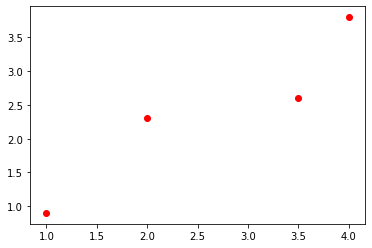$y = kx + b$

$\begin{cases} 1k + b = 0.9 \\ 2k + b = 2.3 \\ 3.5k + b = 2.6 \\ 4k + b = 3.8 \\ \end{cases}$

$y = kx + b\$图例k=1,b=0)$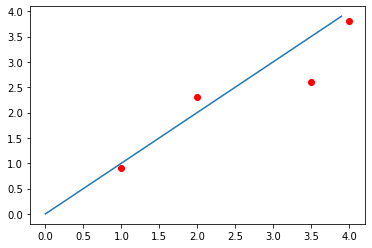因为有些点没有落到直线上，于是我们把误差定义为每一个观测点的y值和我们预测的真实值之间的距离的平方,也就是 $e_i = (f(x_i)-y_i)^2=(kx_i + b - y_i)^2$ 我们的目标是使得总体的误差最小，也就是 $min(\sum_{i=1}^n{e_i})$ 这是一条关于k和b的二元函数，我们求偏导数并找到导数为0的点就可以使其最小，也即是令 $\begin{cases}\frac{\partial{e}}{\partial{k}}=2\sum{(kx_i + b-y_i)x_i} = 0 \\\frac{\partial{e}}{\partial{b}}=2\sum{(kx_i + b-y_i)} = 0 \\\end{cases}$ 我们把原来的方程组写成矩阵的形式(这里把k,b当成待求参数，写成\(x_1,x_2$)

$\begin{bmatrix} 1 & 1 \\ 2 & 1 \\ 3.5 & 1 \\ 4 & 1 \end{bmatrix} \begin{bmatrix} x_1 \\ x_2 \end{bmatrix} =\begin{bmatrix} 0.6\\3\\2.6\\3.8 \end{bmatrix}$

$\begin{bmatrix} e_1\\e_2\\e_3\\e_4 \end{bmatrix} = \begin{bmatrix} 1 & 1 \\ 2 & 1 \\ 3.5 & 1 \\ 4 & 1 \end{bmatrix} \begin{bmatrix} x_1 \\ x_2 \end{bmatrix} -\begin{bmatrix} 0.6\\3\\2.6\\3.8 \end{bmatrix}$

$E =\begin{bmatrix}e_1\\e_2\\e_3\\e_4\end{bmatrix}, A =\begin{bmatrix}1 & 1 \\2 & 1 \\3.5 & 1 \\4 & 1 \end{bmatrix}, X = \begin{bmatrix}x_1 \\ x_2\end{bmatrix}, b = \begin{bmatrix}0.6\\3\\2.6\\3.8\end{bmatrix}$

$E = AX - b$

$E^TE = (AX-b)^T(AX-b)$

$\frac{\partial{E^2}}{\partial{X}}= \frac{\partial{(AX-b)^T(AX-b)}}{\partial{X}}\\= \frac{\partial{(AX-b)^T}}{\partial{X}} *(AX-b)+ \frac{\partial{(AX-b)^T}}{\partial{X}}*(AX-b)\\= 2\frac{\partial{(AX-b)^T}}{\partial{X}}*(AX-b)\\ =2\frac{\partial{(AX)}^T}{\partial{X}}*(AX-b)\\ =2A^T(AX-b)$

$X = (A^TA)^{-1}A^Tb$

$\begin{bmatrix}1 & 1 \\2 & 1 \\3.5 & 1 \\4 & 1 \end{bmatrix}\begin{bmatrix}x_1 \\ x_2\end{bmatrix}=x_1\begin{bmatrix}1 \\2 \\3.5 \\4 \\\end{bmatrix}+x_2\begin{bmatrix}1 \\1 \\1 \\1 \end{bmatrix}=\begin{bmatrix}0.6\\3\\2.6\\3.8\end{bmatrix}$

$v_1 = \begin{bmatrix} 1 \\ 2 \\ 3.5 \\ 4 \\ \end{bmatrix}, v_2 = \begin{bmatrix} 1 \\ 1 \\ 1 \\ 1 \end{bmatrix} b = \begin{bmatrix} 0.6\\3\\2.6\\3.8 \end{bmatrix}$

$ev_1=0,ev_2=0\\ev_1+ev_2=0\\\begin{bmatrix}1\\2\\3.5\\4\end{bmatrix}*e+\begin{bmatrix}1\\1\\1\\1\end{bmatrix}*e=0\\$

$\begin{bmatrix}1&2&3.5&4\\1&1&1&1\end{bmatrix}e=0\\$

$A^T(AX-b)=0$

## 拟合的结果

### 一次拟合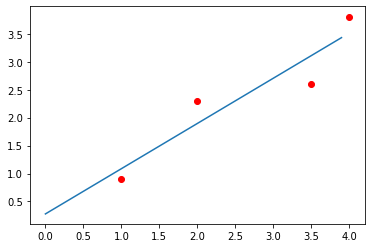###二次拟合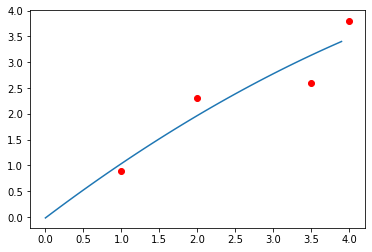###三次拟合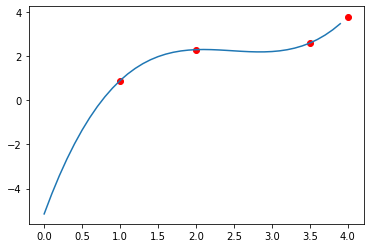##代码(Python)
# -*- coding: utf-8 -*-
"""
Created on Mon Feb 17 13:51:43 2020

@author: urahyou
"""
import numpy as np
import matplotlib.pyplot as plt

x = np.array([3.0, 5, 6, 8, 10])
y = np.array([5.0, 2, 1, 2, 4])

p1 = plt.scatter(x,y,c='red')

def LSD(x, y, n):
N = x.size  #获取方程组个数
A = np.ones(N)

for i in range(1, n+1):
A = np.vstack((A,x**i))  #垂直拼接
A = A.T  #转置回来
#求解
B = np.linalg.inv(A.T@A)@A.T
#求出解系数
sol = np.dot(B, y)
return sol

def poly(x,sol):
y =  np.zeros_like(x)  #每一个x,对应一个y
n = sol.size
for i in range(n):
y += sol[i]*x**i
return y

sol = LSD(x,y,2)
X = np.arange(0, 14, 0.1)
Y = poly(X,sol)
p2 = plt.plot(X,Y)


posted @ 2020-02-23 14:40  裏表異体  阅读(68)  评论(0编辑  收藏